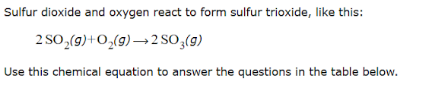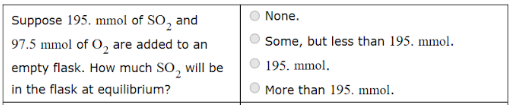# Sulfur dioxide and oxygen react to form trioxide, like this: 2 SO2(g) + O2(g) → 2 SO23(g) Use this chemical equation to answer the questions in the table below. Suppose 195. mmol of SO2 and 97.5 mmol of O2 are added to an empty flask. How much SO2 will be in the flask at the equilibrium?